# Biggest Reuleaux Triangle inscribed within a square inscribed in a semicircle in C?

A Reuleaux triangle is a shape formed by using intersection of three circle, in such a way that each having its center on the boundary of the other two circles. Its boundary is a curve of constant width, the simplest and best known such curve other than the circle itself. Constant width means that the separation of every two parallel supporting lines is the same, independent of their orientation. Because all its diameters are the same.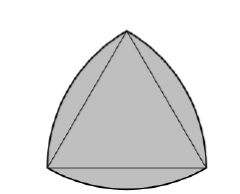The boundary of a Reuleaux triangle is a constant width curve based on an equilateral triangle. All points on a side are equidistant from the opposite vertex.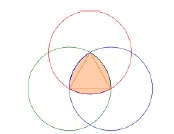To construct a Reuleaux triangle

## Formula for Reuleaux triangle,

Area of the Reuleaux Triangle, if curve based on an equilateral triangle and side of triangle is h

A = (π * h2) / 2 – 2 * (Area of equilateral triangle) = (π – √3) * h2 / 2 = 0.70477 * h2

Biggest Reuleaux Triangle inscribed within a square inscribed in a semicircle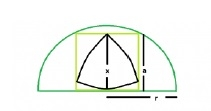if Biggest Reuleaux Triangle inscribed within a square inscribed in a semicircle then it will look like the above image.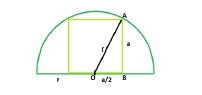if Largest square inscribed in a semicircle then it will look like above image

r be the radius of the semicircle & a be the side length of the square.

The right angled triangle AOB

a2 + (a/2)2 = r2

5*(a2/4) = r2

a2 = 4*(r2/5) i.e. area of the square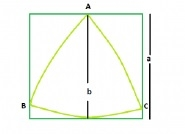Biggest Reuleaux Triangle within A Square

Area of Reuleaux Triangle is 0.70477 * b2 where b is the distance between the parallel lines supporting the Reuleaux Triangle.

distance between parallel lines supporting the Reuleaux Triangle = Side of the square i.e. a

Area of the Reuleaux Triangle, A = 0.70477 * a2

Input:x = 5
Output: 14.0954

## Explanation

Given here is a semicircle of radius r which inscribes a square which in turn inscribes a reuleaux triangle. find the maximum possible area of this reuleaux triangle.

The side of the square inscribed within a semicircle is, a = 2r/√5

x = a.

x = 2*r/√5

Area of Reuleaux Triangle −

A = 0.70477*x^2 = 0.70477*(r^2/5)

## Example

#include <stdio.h>
#include <math.h>
int main() {
float r = 5;
float x = (2 * r) / sqrt(5);
float A = 0.70477 * pow(x, 2);
printf("The area is %f",A);
return 0;
}

## Output

The area is 14.095401